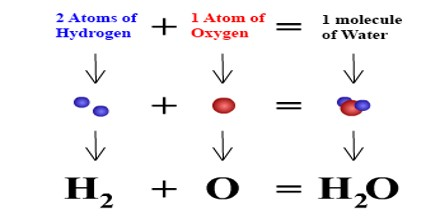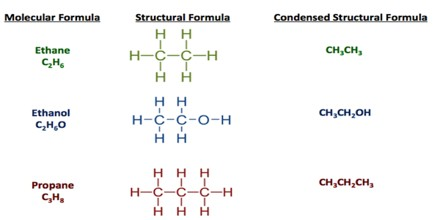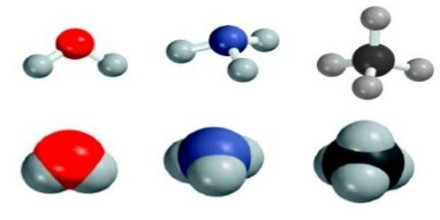Chemical Formula

Definition

Chemical Formula is a way of expression, which states the number and type of atoms present in a molecule of a substance. A chemical formula is not a chemical name, and it contains no words. Although a chemical formula may imply certain simple chemical structures, it is not the same as a full chemical structural formula. Chemical formulas can fully specify the structure of only the simplest of molecules and chemical substances, and are generally more limited in power than are chemical names and structural formulas.A compound is a substance made up of a definite proportion of two or more elements. A chemical formula tells us the number of atoms of each element in a compound. It contains the symbols of the atoms of the elements present in the compound, as well as how many there are for each element in the form of subscripts.

Since a chemical formula must be expressed as a single line of chemical element symbols, it often cannot be as informative as a true structural formula, which is a graphical representation of the spatial relationship between atoms in chemical compounds. It may be used in chemical equations to describe chemical reactions and other chemical transformations, such as the dissolving of ionic compounds into solution.

Examples of Chemical Formula –

There are 6 C atoms and 14 H atoms in a hexane molecule, which has a molecular formula of C6H14.

The chemical formula of table salt or sodium chloride is NaCl. There is one sodium atom and one chlorine atom in each molecule.Types of Chemical Formula

There are different types of chemical formulas and each type gives us different information about a chemical substance. The different types of chemical formulas include: molecular, empirical, structural and condensed structural formulas.

Molecular Formula –

Molecular Formula also known as the “true formula”, the molecular formula states the actual number of atoms of the elements in a single molecule. For example, the molecular formula of the sugar glucose is C6H12O6.

Empirical Formula –

The word empirical is defined as something that is verified by observation. It is the simplest ratio of the whole number of elements in a compound. It’s sort of like simplifying mathematical fractions. Sometimes the molecular and empirical formula are the same (e.g., H2O), while other times the formulas are different. For example, the empirical formula of glucose is CH2O, which is obtained by dividing all of the subscripts by the common value (6, in this case).

Structural Formula –

The structural formula shows both the actual number of atoms of elements in a compound, how the atoms are arranged as well as which atoms are bonded to one another. A structural formula shows the chemical bonds. There are different types of structural formulas, too. Some indicate the two-dimensional structure, while others describe the three-dimensional arrangement of atoms. For example, ethanol (grain alcohol people can drink) and dimethyl ether (a toxic compound) share the same molecular and empirical formulas.

Condensed Formula –

This type of chemical formula is a sort of shorthand notation; the condensed structural formula may omit the symbols for carbon and hydrogen in the structure, simply indicating the chemical bonds and formulas of functional groups. The written condensed formula lists the atoms in the order in which they appear in the molecular structure. For example, the molecular formula of hexane is C6H14, but its condensed formula is CH3(CH2)4CH3. This formula not only provides the number and type of atoms, but also indicates their position in the structure.Hill System or Hill Notation

The Hill System also known as Hill Notation, is a system of writing empirical chemical formulas, molecular chemical formulas and components of a condensed formula such that the number of carbon atoms in a molecule is indicated first, the number of hydrogen atoms next, and then the number of all other chemical elements subsequently, in alphabetical order of the chemical symbols. When the formula contains no carbon, all the elements, including hydrogen, are listed alphabetically.

It is the most commonly used system in chemical databases and printed indexes to sort lists of compounds.

Examples: The following formulas are written using the Hill system, and listed in Hill order:

• BrI
• CCl4
• CH3I
• C2H5Br
• H2O4S